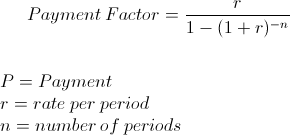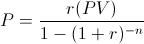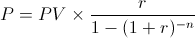# Annuity Payment Factor - PV

Annuity Payment Factor (PV) Calculator (Click Here or Scroll Down)The annuity payment factor is used to simplify calculations for an annuity payment. The formula shown is specifically for simplifying annuity payment calculations when the present value of the annuity is known(in contrast to the future value being known).

As with any financial formula that involves a rate and term, it is important to match the payment periods with the rate per period in the annuity payment factor formula. For example, if the payments are made or received monthly, then the rate needs to be the effective monthly rate.

## Use of the Annuity Payment Factor When Present Value Is Known

As previously stated, the annuity payment factor is used to allow for quick calculations when an individual is figuring the payment on an annuity when present value is known. This is accomplished by using an annuity payment factor table to find the factor for a given rate and number of periods. The actual periodic payment of an annuity can then be determined by multiplying the present value, or original amount, by the annuity payment factor.

For example, suppose that an individual is wanting to calculate the monthly payments on an original balance of \$2,000, to be paid off in 2 years based on a rate of 6% compounded monthly. By looking at the annuity payment factor table which uses the formula at the top of this page, the annuity payment factor of 24 months at a rate of .5% per month(6% per year) is .04432. This annuity payment factor found on the table can then be multiplied by the present value of \$2,000 which would return a monthly payment of \$88.64.

## How is the Annuity Payment Factor Derived?

The annuity payment factor formula using present value can be found by factoring out present value from the annuity payment formula. The annuity payment formula shown on this site isThe annuity payment formula shown above can be rewritten asBy factoring out present value from the annuity payment formula, the process of calculating the payment can be simplified by creating a table of factors using the formula shown at the top of the page with various terms and rates.

New to Finance?